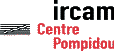Serveur © IRCAM - CENTRE POMPIDOU 1996-2005.Tous droits réservés pour tous pays. All rights reserved.

# One and Two Mass Model Oscillations for Voice and Instruments

Xavier Rodet

## Abstract:

Two movements seem possible for the lips of the trumpet player, upward and outward striking . A basic model, using one or the other movement, is proposed so that its behavior can more easily be understood and controlled. The two movements are compared. The model appears as a dynamic system of two loops coupled by a nonlinear function, one loop describes the dynamics of the single mass and the other represents the acoustic wave in the bore. Being much simpler, this model is simulated in real time on a workstation, allowing for easy experimentation of different designs and parameter values.

## I. Introduction

Physical models consisting of an instantaneous nonlinearity and a linear feedback loop are relatively easy to understand and control [Rodet 93a]. But in the case of the trumpet or of the voice, the assumption of a massless reed, hence of an instantaneous nonlinearity, cannot be maintained. The movement of the lips of the trumpet player is discussed for instance in [Yoshikawa 95]. It seems that two movements are possible, as a function of several parameters such as the register and the shape of the bore. The first movement is orthogonal to the flow - it is called upward striking - and is comparable to the movement of the vocal cords. In the second movement, the lip moves essentially back and forth - it is called outward striking . In [Rodet 92], we proposed a physical model and simulation of the trumpet, of the lips of the trumpet player and of the interaction between the two. The lip was modelled as a rigid parallelepiped with two degrees of freedom acoustically coupled. This leads to a system of five non-linear differential equations. The model exhibited behaviors comparable to natural trumpet playing and produced sounds with typical brass quality. Because of the complexity of the model, we underlined the necessity to devise some playing or control model and concluded that much more work was needed to better analyze the physical processes involved, to understand and represent them in a model simultaneously powerful enough to render all the subtlety of natural sounds, yet simple enough to compute and control. The research presented in this article brings some answer to these questions. In particular we want to test whether one mass is sufficient to render the basic properties of a trumpet oscillation. This allows a much simpler nonlinear dynamical model, so that its behavior can be understood and controlled. This requirement is very important for musical applications as explained in [Rodet 93a & 94a]. In section III, we discuss and compare the force given by [Fletcher 91], the force which [Flanagan 68] uses for the one-mass model of vocal cords and the equations which we developed to implement the model. All these models even if they do not represent a classical musical instrument, are nonlinear dynamical systems which may have interesting musical properties. The simplest model appears as a dynamic system of two loops coupled by a nonlinear function. One loop describes the dynamics of the single mass and the other loop represents the acoustic wave in the bore. The upward and outward striking movements are compared. Being much simpler, this model is simulated in real time on a workstation, allowing for easy experimentation of different designs and parameter values.

## II. One Mass Model

Consider a mass m, the vertical only movement of which can close more or less an acoustic tube (Fig. 1.).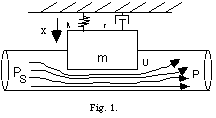Let us call x the vertical position of the mass, A the area of the orifice, r the air density and Ps (s as in subglottal pressure by analogy with vocal cords) the blowing pressure that generates some flow in the tube. We assume a Bernoulli flow in the orifice between the mass and the opposite wall of the tube. Let us first follow the experimental measurements of [van den Berg 57]. We designate by Bernoulli pressure the value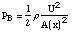Then the acoustic pressure at the inlet (p1) and outlet (p2) of the glottal orifice are: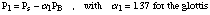,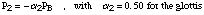Let d be the mass thickness and l the orifice length. The pressure force when the orifice is not closed is [Flanagan 68]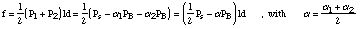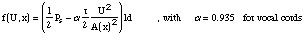But the measurements of [van den Berg 57] are contested [Pelorson & al. 95]. Let us now consider a different hypothesis and assume a jet flow out of the orifice. Then, with sgn(Ps-P) designating the sign of (Ps-P):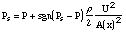If the tube is open to the free atmosphere after the mass, P=0, there is no vertical force on the mass! The jet flow is a simplification which leads to a non interesting case for our simplest one-mass model uncoupled to any tube.
Using [Flanagan 68], we now consider the particular case where the tube opens into the "free atmosphere" after the orifice between the mass m and the opposite wall of the tube. This constitutes a one-mass model not coupled to any resonator. Let us show that the volume velocity U passing through the orifice depends only on the pressure Ps and on the area of the orifice which we suppose to be a function of x only. Therefore the pressure on the mass is a function of x only for a fixed blowing pressure Ps. If the tube opens in the free atmosphere, the equivalent circuit, after [Flanagan 68], is shown on Fig 2.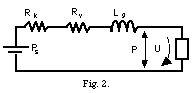Because we are interested only in rather low frequency behavior, let us neglect Lg . Then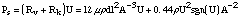Therefore we can write U as a function of Ps and A only. We find the first term @101 and the second @104 for the maximum opening. But for small opening the first term should become comparable or bigger than the second. Finally, the pressure force on the mass m is a function of x for fixed Ps, say f(x) Similarly, when the orifice is closed, the force should depend only on x. As a consequence the equation for the mechanical oscillator is:
(1)
where k>0 is the spring constant and r>0 is the viscous damping. The corresponding state equation is: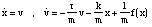It is easy to show that, since the force depends only on x, for r > 0 this system cannot oscillate in the sense that it does not have a periodic trajectory [Rodet & Steinecke 94]. Different possibilities exist to make it oscillate:
1. Have a force term which depends on the velocity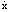. The violin - i.e. bow-string interaction - is an example. It can easily be proved that a one-mass model with a bow-string like force function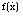has at least one periodic trajectory.
2. Add one dimension to the system by adding a second mass leading to a two-mass model where the two masses are linearly coupled (by a spring) and non linearly coupled by the flow. This is made simple with the assumption of a jet flow through the minimum opening [Steinecke 93]. Such two-mass model can oscillate
3. Add a with memory system (this adds some dimension) to the one mass. For instance a flow-pressure coupling by a linear system, such as an acoustic tube, which can also be considered as a delay term. We now focus on this case. It is simple since it consists of a one dimensional oscillator coupled to a delayed feedback loop [Rodet 93a]. It seems to model some important behavior of trumpet like instruments.

## III. One-mass coupled to an acoustic tube, the simplest trumpet-like model

We consider the same one-mass model as before but with an acoustic tube after the mass, which induces a load on the mass oscillator. The effect of the tube can also be seen as a delay term added to the system. Let P be the pressure in the mouthpiece. The flow U depends on x and also on P as opposite to the uncoupled case studied in the preceding section. Therefore, the pressure force on the mass is a function of x and P, say f(x, P) and the mechanical equation is: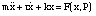In [Fletcher 91]'s notation we have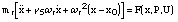Let us now consider which pressure effectively acts on the mass. There are different ways to derive the expressions for the force. We want to discuss and compare the force given by Fletcher (a general equation to describe wind driven instruments), the force which Flanagan uses for the one-mass model of vocal cords and the equations which we developed to implement the model. All these models even if they do not represent a classical musical instrument, are nonlinear dynamical systems which may have interesting musical properties.

### III.1. Fletcher's model

Fletcher considers a mass-movement in the direction of the resulting flow, as shown on Fig. 3. This is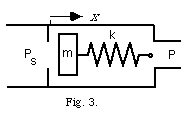associated with a complicated "flow geometry". The resulting force is the sum of a flow independent term which displaces the mass even if there is no flow and a flow dependent term (a modified Bernoulli pressure). The "static", flow-independent, force is very approximately proportional to the pressure difference Ps-P, because on the left side we have Ps and on the right side we have P. In detail, the situation is more complicated. This force is diminished by a flow dependent term. For the idealized case this term is proportional to the Bernoulli pressure PB. The force is then in Fletcher's notation: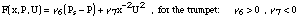To calculate the volume flow velocity Fletcher uses the empirical equation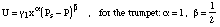The parameters used for the trumpet correspond to a kinetic-only resistance in the orifice. With this parameters the equation for the force results into: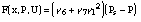Remark: Because of PB U2/X2 the Bernoulli pressure would not necessarily go to zero if x Æ 0. This behavior would be associated with a discontinuity in the force at x = 0. But a > 1 includes the increased viscous resistance for small gaps. Then PB Æ 0 when l Æ 0

### III.2 Flanagan's model

The movement of the mass is perpendicular to the direction of the flow. Pressures are respectively Ps before the mass and P after the mass. To calculate the acting pressure Flanagan uses the measurements of [van den Berg 57] and assumes a linear pressure change over the thickness of the mass. As we have seen in section I, the resulting force is: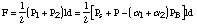For calculating U, Flanagan considers the pressure drop over a viscous resistance and a kinetic resistance. Here we neglect the inductance which describes the acceleration of the flow.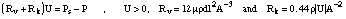For large opening the term Rk is greater than the term Rv and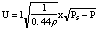For the resulting force we get: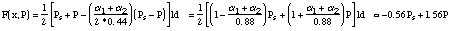## IV. Implementation

The geometry and the movement of the mass are considered as in the model of Flanagan, but the pressure is considered as constant over the mass and equal to the pressure before the mass Ps diminished by a modified Bernoulli pressure. The flow before the orifice is neglected and a jet of the same cross section as the orifice is assumed after the mass. Then we get a force: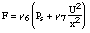where g6 and g7 correspond to Fletcher's notation. From our point of view g6 = ld as in Flanagan's model. For an idealized Bernoulli flow and for Ps-P>0 we get: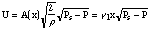To include other losses we can use a parameter g1 as Fletcher does. Since Ps-P can be positive or negative,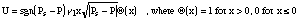(2)
The resulting force is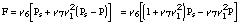Remark: Only the combination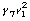is used. Additional losses could be considered. To make the system as simple as possible, an idealized flow is assumed, which leads to F = ldP: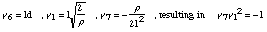## V. Comparison of the models

The model implemented is more or less equivalent to the model of Flanagan. We have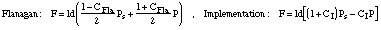where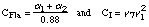are constants of the corresponding models.
The resulting forces are equal when: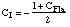Setting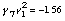in the implemented model leads to Flanagan's model. Fletcher's model is really different, because F = CFle(Ps-P) cannot be transformed into the other models by setting parameters. Therefore, assuming that there is a motion in the direction of the flow, which is coupled to the perpendicular motion [Rodet 92], we choose to add an empirical term which is proportional to the pressure difference Ps-P. Then we have the complete pressure function for the open case: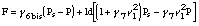(3)
When the lips are closed there is no flow and the flow dependent term vanishes leaving only the force which results from the coupling to the pressure difference. During the impact we add an additional restoring force which is a linear function of x. The corresponding spring constant is c = 3k. The equation of the mechanical oscillator is therefore: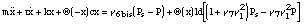(4)

## VI. Coupling of the mass and the tube

To make things as simple as possible, we reduce the bore to a cylindrical tube. We then follow the formulation of [McIntyre et al. 83]: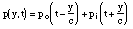and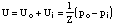where y is the direction of the planar wave, the pressure p is the sum of the outgoing pressure po and the incoming pressure pi, c is the sound velocity and the volume flow U at y=0, i.e. at the lips position, is the sum of the flow of the outgoing and of the incoming waves. The volume flow velocity and the pressure are coupled by the acoustical impedance in the tube Z = rc/Acyl where r is the air density and Acyl is the cross sectional area of the tube. The pressure pi is calculated as a convolution of the outgoing pressure po with a reflection function r(t):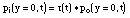The reflection function r(t) can be formulated so as to make explicit the delay T = 2L/c corresponding to one round trip when L is the effective length of the tube. To get a simple model we often use only a reflection coefficient designated by reflect: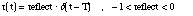Finally, the pressure p is: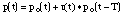The acoustic tube is then coupled to the mechanical oscillator by the given equations for the pressure P and the volume flow velocity U. The relationship for the Bernoulli flow and the relationship for the wave in the tube are to be taken in consideration. Following [McIntyre et al. 83], values for P and U are obtained by solving the following system of three equations: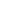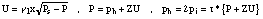of variables P, U and ph. Then the system is solved by iteration. We take a given x and ph as the incoming pressure wave which is supposed to be not influenced by the outgoing wave. Knowing ph we get U and P, these values are used to calculate the acting force on the mass and solve the difference equation for x. Note that U and P depend on x which is taken as a parameter to solve the equation and calculate the force. In the implementation we have: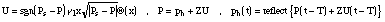For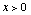we get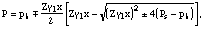upper sign for Ps>ph, lower for Ps<ph,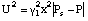For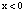we get: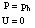The graphical solution of U and P is shown in Fig. 4. For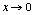the curve 1 reaches the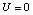axis and the curve 2 results into P = ph. We can interpret the system as a circuit which consists of two loops. One loop describes the dynamics of the mechanical oscillator. For a known pressure P we can solve the differential equation for x which we use to calculate P which also depends on the evaluation of the acoustic wave in the tube.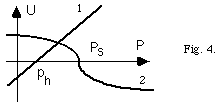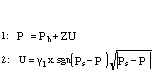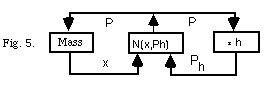This is represented by the second loop which gives the pressure of the incoming wave ph=2pi. Both loops are coupled by a nonlinear function to calculate P(x,Ph) and U(x, Ph) respectively. Finally, this can be simplified in P alone (Fig. 5).We think that this system captures an important part of the behavior of trumpet-like instruments in the interaction of the modes of the tube loop with the own resonance of the mass subsystem [Rodet 92].

## VII. Discussion of parameters

All values which are given here are in units of the SI system. Air density is r = 1.13 and sound velocity in air is c = 440.

### Geometry of the lips

These values were chosen to be in the order of the real one and the bevavior of the system does not seem to be very sensitive to precise values, but the influence of variations in this range needs more investigation.
Length of the lip: l= 0.01, thickness of the lip: d = 0.005. Values used for the 2-mass model of the vocal cords are smaller, the vibrating part of the vocal cords has a thickness of d = 0.003.

### Elastic properties of the lips

We take as standard for the mass m the value given by [Strong 90] and chose the spring constant to the corresponding natural frequency given by Fletcher (w0 = 3100). The damping is set to get a quality factor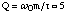.
Mass of the lip: m0 = 1.5.10-4, stiffness of the lip: k0 = 1400, additional stiffness during closure c0 = 3k, damping constant r0 = 0.1. The natural frequency of the lips fLips is varied by using a tension factor tension which scales the elastical properties in the following way.
m = m0/tension, k = k0.tension, c = 3k, r = r0. Thus, tension gives the scaling of fLips.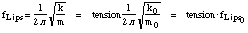By keeping r constant, the Q-factor does not change. In the program we can use fLips as parameter and scale m, k, c and r in the given way. But then the damping factor r/2m becomes small for lower frequencies. We can otherwise keep r/2m constant by scaling r as r = r0/tension. This scaling results in more difficult playing of lower modes. The value of r is not only important for oscillation onset, but also for the stability of modes. Because r influences the frequency of the lips, it is possible to enter another mode by an independent change of r.

### Properties of the tube

The resonator is assumed to be a cylindrical tube of length L and cross sectional area Acyl. From this geometry related parameters we get the acoustical properties: the acoustical impedance Z and the resonance frequency fTube.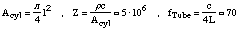For the reflection at the open end we use: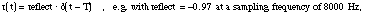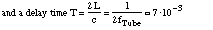According to Fletcher the upper limit of the mouth pressure is around 10 kPa.
Empirical parameters

As mentioned above we assume an idealized flow and use the parameter6scale to represent the ratio of the force which is parallel to the flow to that which is orthogonal to the flow: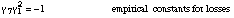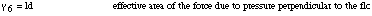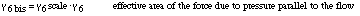Then from equation 3 in section V the force term becomes: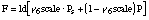,
Finally, to avoid a discontinuity in the force term, we use the function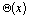, (see equation 2 of section IV) in the right term also, as already written in section V: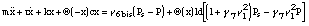### Discussion of6scale

By changing6scale,the dynamics of the system can be significantly influenced. For6scale = 0, F increases linearly with P. If6scale = 1, F is proportional to ldPs. For6scale > 1 the coefficient in P becomes negative, i.e. the higher pressure the smaller force. Thus, for6scale < 1 a high pressure in the tube causes a strong force on the mass and for6scale > 1 the force becomes smaller and can become negative for large P. Additionally to the discussed cases, the flow independant force of the Fletcher model is approached for6scale > 1.
This different relationship between F and P leads to a different dynamic behavior. If F increases with P, the mass follows the pressure, because the maximum of P corresponds to the maximal force leading to a maximum of the displacement. For6scale > 1 the minimum of P leads to the maximum of F. In this case the displacement of the mass precedes the pressure which is more typical for the real trumpet. Nevertheless, waveforms are in both cases nearly the same. We have found that the model is easy to play for higher values6scale , i.e. 2.0, which corresponds to the outward striking. model.

## VIII. Conclusion

We have implemented the systems described here above in HTM [Freed 92] allowing for real-time synthesis and experimentation with various parameter and design choices. The model behavior appears clearly connected to natural trumpet playing and the sounds have some typical characteristics of brass quality, in the transient at least since the model is extremely far from any natural instrument. The simplest model appears as a dynamic system of two loops coupled by a nonlinear function. One loop describes the dynamics of the single mass and the other implemented with a delay, represents the acoustic wave in the bore. This is to be compared with a basic clarinet model [Rodet 93b] which consists of one delayed loop only and a basic flute model [Rodet 95] which consists of two delayed loops without any mass. We are now testing and comparing the different configurations. Even though Flanagan's and Fletcher models in our implementation can oscillate on several modes of the tube, the second is easier to play and offers a larger range of playing values. We are also experimenting the behavior of the system in terms of stability, oscillation, number of solutions and their stability, as well as the influence of parameter values on the control of the system, such as speed of transients. We are also trying other bore shapes and approximations, in particular to take into account the cup and the bell of a trumpet. More details will be given at the conference as well as sound examples.

### Acknowledgements

We thank Ina Steinecke who did a great part of this work and C. Vergez and N. Misdariis [Misdariis 95] for many useful discussions and comments.

#### References

[Martin 42] D. W. Martin, Lip vibrations in a cornet mouthpiece, J. Acoust. Soc. Am. 13 , 305-308, 1942
[van den Berg 57] J. W. van den Berg, J. T. Zantema, and P. Doornenbal, Jr., On the air resistance and the Bernoulli effect of the human larynx, J. Acoust. Soc. Am. 29 , 626-631, 1957
[Flanagan 68] J. L. Flanagan and L. L. Landgraf, Self-oscillating source for vocal-tract synthesizers, IEEE Trans. Audio and Electroacoustics, AU-16, 57-64, 1968
[Ishizaka 72] K. Ishizaka and J. L. Flanagan, Synthesis of voiced sounds from a two-mass model of the vocal cords, Bell. Syst. Techn. J. 51 , 1233-1268, 1972
[Benade 76] Benade (A.H.) -- Fundamentals of Musical Acoustics (New York, Oxford Univerty Press,1976).
[McIntyre 83] M. E. McIntyre, R. T. Schumacher and J. Woodhouse, On the oscillations of musical instruments, J. Acoust. Soc. Am. 74 (5), 1325-1345, 1983
[Smith 86] Smith, J.O., "Efficient simulation of the reed-bore and bow-string mechanism", Proc 1986 Int. Computer Music Conf., P. Berg, eds., Computer Music Assoc., San Francisco, pp. 275-280, 1986.
[Titze 89] I.R. Titze, A four parameter model of the glottis and vocal fold contact area Speech, Commmuniction 8, 191-201.
[Rodet 90] Rodet, X., Depalle Ph., "Modèles de Signaux et Modèles Physiques d'Instruments", Proc. of the Colloqu. on Physical Modeling, Genoble, France, October 1990 Editions de la Maison de Sciences de l'Homme, Paris, France, 1992.
[Strong 90] W. J. Strong, Computer simulation of a trumpet, J. Acoust. Soc. Am. Suppl. 1 87 , S138 (1990).
[Fletcher 91] N. H. Fletcher and T. D. Rossing, The Physics of Musical Instruments, Springer-Verlag New-York 1991
[Cook 92] Cook, P., "A meta-wind-instrument physical model", Proc. International Computer Music Conference, San Jose, pp. 273-276, Oct. 1992.
[Freed 92] A. Freed, "Tools for Rapid Prototyping of Music Sound Synthesis Algorithms and Control Strategies", in: Proc. Int. Comp. Music. Conf., San Jose, CA, USA, Oct. 1992
[Keefe 92] Keefe, D., "Physical Modeling of Wind Instruments", Computer Music Journal, MIT Press, Vol 16 No. 4, pp. 57-73, Winter 1992.
[Matignon 92] Matignon, D., Depalle, Ph., Rodet, X., "State space models for wind-instrument synthesis", Proc. International Computer Music Conference, San Jose, pp. 273-276, Oct. 1992.
[Modèles 92] "Modèles Physiques, Création Musicale et Ordinateurs ", Proceedings of the Colloq. on Phys. Modeling, ACROE, Genoble,France, Oct. 1990, Editions de la Maison de Sciences de l'Homme, Paris, 1992.
[Rodet 92] Rodet, X., Depalle Ph., "A physical model of lips and trumpet", Proc. International Computer Music Conference, San Jose, pp. 132-135, Oct. 1992.
[Vössing 92] H. Vössing, Experimentelle Untersuchungen zur Physik der Trompete, Diplomarbeit, Johann Wolfgang Goethe - Universität Frankfurt am Main, 1992
[Rodet 93a] Rodet, X., "Flexible yet Controllable Physical Models: a Nonlinear Dynamics Approach", Rodet, X., Proc. Int. Computer Music Conference, Tokyo, 10-15 Sept. 1993.
[Rodet 93b] Rodet, X., "Models of Musical Instruments from Chua's Circuit with Time Delay", IEEE Trans. on Circ. and Syst., Special Issue on Chaos in nonlinear electronic circuits, Sept. 1993.
[Steinecke 93] I. Steinecke, Untersuchungen an einem vereinfachten Stimmlippenmodell, Diplomarbeit, Humboldt-Universität, 1993
[Rodet 94a] Rodet, X., "Stability/Instability of Periodic Solutions and Chaos in Phyical Models of Musical Instruments", Proc Int. Computer Music Conference, Copenhaguen, Sept. 1994.
[Rodet 94b] Rodet, X., Steinecke, I., "One and two mass models oscillations for voice and instruments", unpublished internal report, IRCAM, March 1994.
[Verge 94] Verge, M.P., "Jet Oscillations and jet drive in recorder-like instruments", Acta Acustica 2 (1994), pp 403-419.
[Pelorson & al. 95] Perlorson, X., Hirschberg, A., Wijnands, A.P.J., Description of the flow through in-vitro models of the glottis during phonation,
[Rodet 95] Rodet, X., "Basic structure and real-time implementation of J.M. Verge's flute model", unpublished internal report, IRCAM, Mai 1995.
[Misdariis 95] N. Misdariis and C. Vergez, Modèle de base du fonctionnement des instruments excités par une lèvre vibrante, rapport de DEA, IRCAM, Juin 1995.

____________________________
Server © IRCAM-CGP, 1996-2008 - file updated on .

____________________________
Serveur © IRCAM-CGP, 1996-2008 - document mis à jour le .